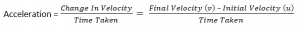Thursday , July 18 2019

# Kinematics

### Distance:

It is defined as the total path length taken between two points. It is a scaler quantity. Its SI Unit is metre, m.

### Displacement:

It is defined as the change of position of a point in a particular direction. It is a Vector quantity as it has both magnitude and direction. Its SI unit is also  metre, m.

Remember:

If an object is moving in one direction along a straight path, there is no difference between distance and displacement.

If an object is under a circular motion. It completes one resolution and goes back to the starting point. The displacement is zero but the distance travelled is the circumference of the circular path.

### Instantaneous Speed:

Instantaneous speed is a speed of a particular instance of time .

### Uniform Speed:

Equal distances covered in equal intervals of time gives us uniform speed.

*Remember if an object is under circular motion, its speed is uniform but the velocity is changing. It is   because the direction of motion keeps changing when the object is moving.

Difference Between Velocity And Speed:

Velocity has magnitude and direction while speed has magnitude only.

### Acceleration:

Acceleration is defined as the rate of change of velocity.SI unit : metre per second , ms-1

### Acceleration Due To Gravity:

The force of gravity pulls down all objects here on earth. If objects are allowed to fall , they accelerate downwards. If there is no air resistance or friction, all objects will fall with the same acceleration. The acceleration is called the acceleration due to gravity, which is always pointing downwards.

The acceleration due to gravity is 10 ms -2.

• Free fall of objects under gravity in the absence of air resistance has a constant acceleration.
• Free fall of objects under gravity in the presence of air resistance has decreasing acceleration.## Turning Effects Of Forces

Moments: The moment of a force is the product of the force and the perpendicular …

### One comment

1.Thank U So Much For That